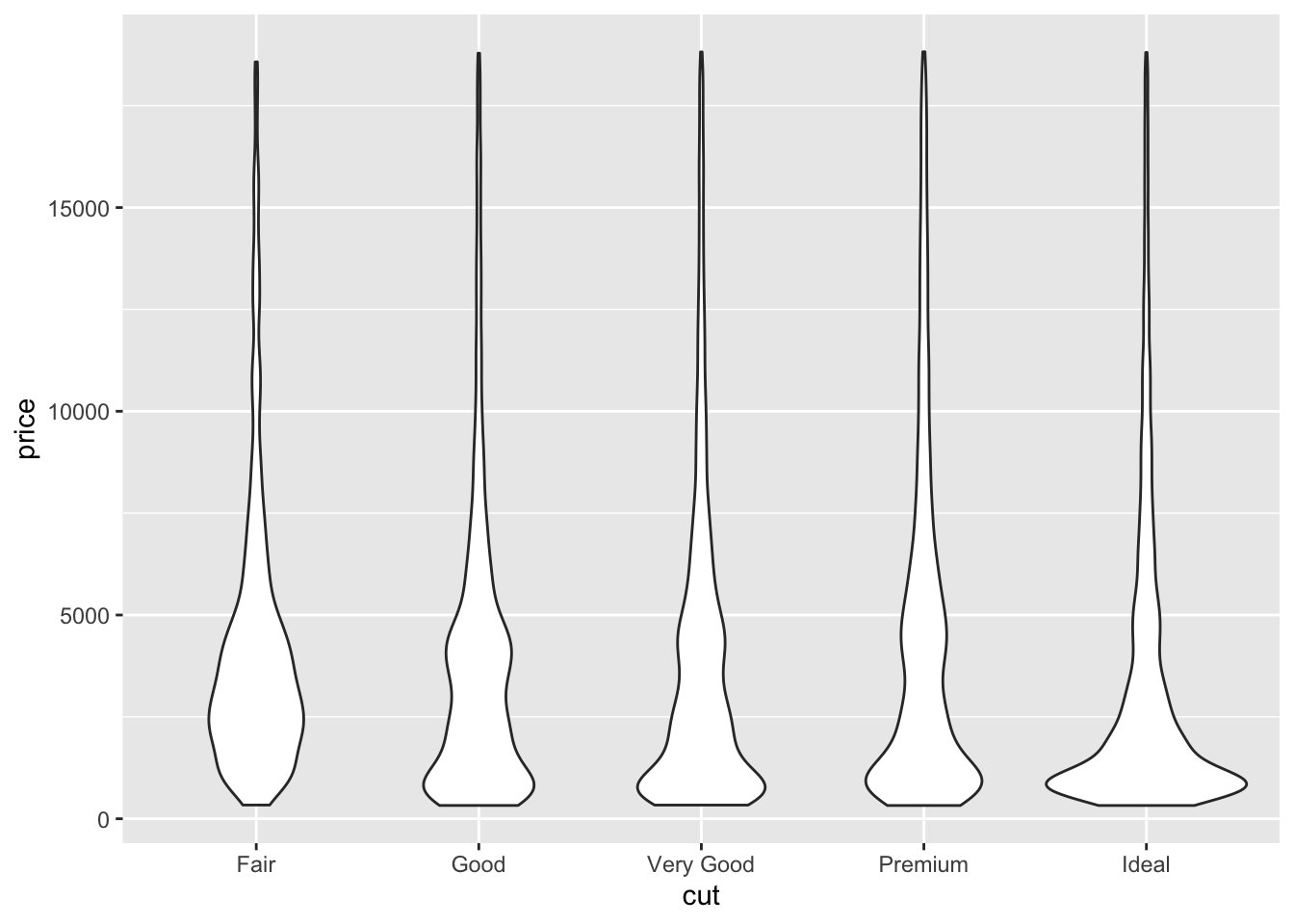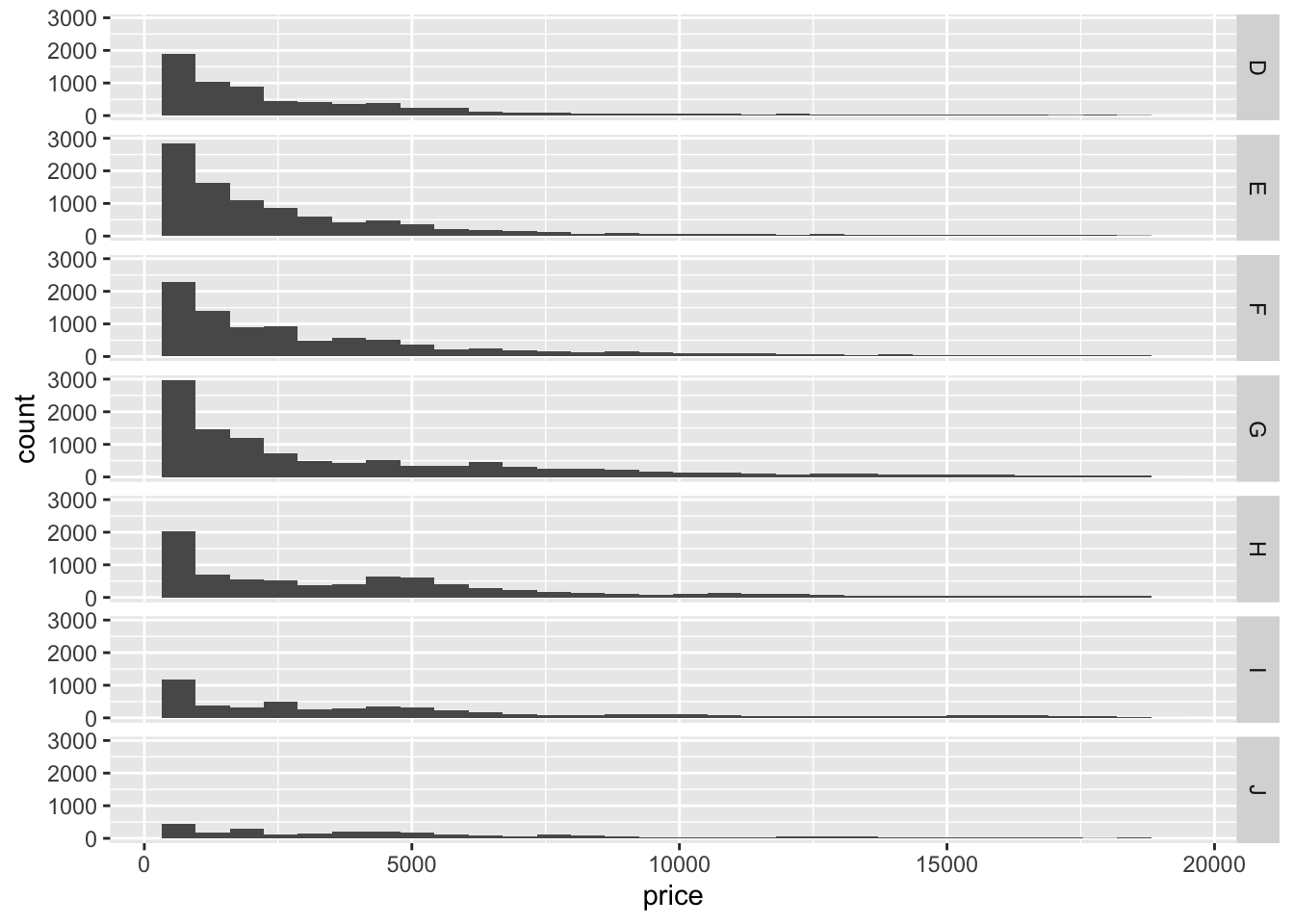library(tidyverse)
## ── Attaching packages ───────────────────────────────────────── tidyverse 1.2.1 ──
## ✔ ggplot2 3.2.1     ✔ purrr   0.3.3
## ✔ tibble  2.1.3     ✔ dplyr   0.8.3
## ✔ tidyr   1.0.0     ✔ stringr 1.4.0
## ✔ readr   1.3.1     ✔ forcats 0.4.0
## ── Conflicts ──────────────────────────────────────────── tidyverse_conflicts() ──
## ✖ dplyr::lag()    masks stats::lag()

### Problems

We’ll begin by doing all the same data processing as in lecture.

# Load data from MASS into a tibble
birthwt <- as_tibble(MASS::birthwt)

# Rename variables
birthwt <- birthwt %>%
rename(birthwt.below.2500 = low,
mother.age = age,
mother.weight = lwt,
mother.smokes = smoke,
previous.prem.labor = ptl,
hypertension = ht,
uterine.irr = ui,
physician.visits = ftv,
birthwt.grams = bwt)

# Change factor level names
birthwt <- birthwt %>%
mutate(race = recode_factor(race, 1 = "white", 2 = "black", 3 = "other")) %>%
mutate_at(c("mother.smokes", "hypertension", "uterine.irr", "birthwt.below.2500"),
~ recode_factor(.x, 0 = "no", 1 = "yes"))

#### 1. Some table practice

(a) Create a summary table showing the average birthweight (rounded to the nearest gram) grouped by race, mother’s smoking status, and hypertension.

bwt.summary <- birthwt %>%
group_by(race, mother.smokes, hypertension) %>%
summarize(mean_bwt = round(mean(birthwt.grams), 0))

(b) How many rows are there in the summary table? Are all possible combinations of the three grouping variables shown? Explain.

There are 11 rows. This does not reflect all possible combinations. In particular, we see that no row is shown for smoking other race mothers with hypertension.

(c) Repeat part (b), this time adding the argument .drop = FALSE to your group_by() call. What happens?

birthwt %>%
group_by(race, mother.smokes, hypertension, .drop = FALSE) %>%
summarize(mean_bwt = round(mean(birthwt.grams), 0))
## # A tibble: 12 x 4
## # Groups:   race, mother.smokes 
##    race  mother.smokes hypertension mean_bwt
##    <fct> <fct>         <fct>           <dbl>
##  1 white no            no               3436
##  2 white no            yes              3100
##  3 white yes           no               2819
##  4 white yes           yes              2918
##  5 black no            no               2813
##  6 black no            yes              3142
##  7 black yes           no               2656
##  8 black yes           yes              1135
##  9 other no            no               2875
## 10 other no            yes              2063
## 11 other yes           no               2757
## 12 other yes           yes               NaN

#### 2. Plotting the diamonds data

(a) Construct a violin plot of showing how the distribution of diamond prices varies by diamond cut.

ggplot(data = diamonds, aes(x = cut, y = price)) +
geom_violin()(b) Use facet_grid with geom_historam to construct 7 histograms showing the distribution of price within every category of diamond color.

ggplot(data = diamonds, aes(x = price)) +
geom_histogram() +
facet_grid(color ~ .)
## stat_bin() using bins = 30. Pick better value with binwidth.